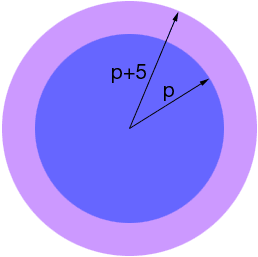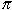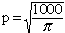Patrick secondary (math 20) Student Question : The community of melfort is planning to build a circular wading pool in the park. The pool will cover an area of 1000m2. The building committee has decided to put a 5m cement pad around it. How much additional area will the cement pad take up? (remember A=pi r squared) Hi Patrick, A diagram here helps a lot.The pool has an unknown radius of p meters and hence the radius to the outside edge of the cement is p + 5 meters. Since the area of a circle of radius r isr2, the area of the cement pad is(p + 5)2 -p2 All that remains is to find p and then perform the calculation. Since the area of the pool is 1000 m2 we know that 1000 =p2 ThusI hope this helps, Harley Go to Math Central